×#### Thank you for registering.

One of our academic counsellors will contact you within 1 working day.

Click to Chat

1800-1023-196

+91-120-4616500

CART 0

• 0

MY CART (5)

Use Coupon: CART20 and get 20% off on all online Study Material

ITEM
DETAILS
MRP
DISCOUNT
FINAL PRICE
Total Price: Rs.

There are no items in this cart.
Continue Shopping• Complete JEE Main/Advanced Course and Test Series
• OFFERED PRICE: Rs. 15,900
• View Details

```Chapter 10: Congruent Triangles Exercise – 10.6

Question: 1

In ΔABC, if ∠A = 40° and ∠B = 60°. Determine the longest and shortest sides of the triangle.

Solution:

Given that in ΔABC, ∠A = 40° and ∠B = 60°We have to find longest and shortest side

We know that,

Sum of angles in a triangle 180°

∠A + ∠B + ∠C = 180°

40° + 60° + ∠C = 180°

∠C = 180° - ((10)0°) = 80°

∠C = 80°

Now,

⟹ 40° < 60° < 80° = ∠A < ∠B < ∠C

⟹ ∠C is greater angle and ∠A is smaller angle.

Now, ∠A < ∠B < ∠C

⟹ BC < AAC < AB [Side opposite to greater angle is larger and side opposite to smaller angle is smaller]

AB is longest and BC is smallest or shortest side.

Question: 2

In a ΔABC, if ∠B = ∠C = 45°, which is the longest side?

Solution:

Given that in ΔABC,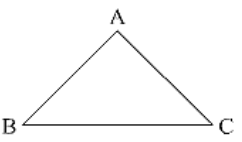∠B = ∠C = 45°

We have to find longest side

We know that.

Sum of angles in a triangle =180°

∠A + ∠B + ∠C = 180°

∠A + 45° + 45° = 180°

∠A = 180° - (45° + 45°) = 180° - 90° = 90°

∠A = 90°

Question: 3

In ΔABC, side AB is produced to D so that BD = BC. If ∠B = 60° and ∠A = 70°. Prove that: (i) AD > CD (ii) AD > AC

Solution:

Given that, in Δ ABC, side AB is produced to D so that BD = BC.

∠B = 60°, and ∠A = 70°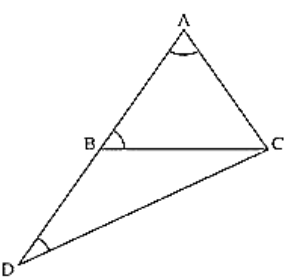To prove,

First join C and D

We know that,

Sum of angles in a triangle =180°

∠A + ∠B + ∠C = 180°

70° + 60° + ∠C = 180°

∠C = 180° - (130°) = 50°

∠C = 50°

∠ACB = 50° ... (i)

And also in Δ BDC

∠DBC =180 - ∠ABC [ABD is a straight angle]

180 - 60° = 120°

and also BD = BC[given]

∠BCD = ∠BDC [Angles opposite to equal sides are equal]

Now,

∠DBC + ∠BCD + ∠BDC = 180° [Sum of angles in a triangle =180°]

⟹ 120° + ∠BCD + ∠BCD = 180°

⟹ 120° + 2∠ BCD = 180°

⟹ 2∠BCD = 180° - 120° = 60°

⟹ ∠BCD = 30°

⟹ ∠BCD = ∠BDC = 30° .... (ii)

∠BAC ⟹ ∠DAC = 70° [given]

∠BDC ⟹ ∠ADC = 30° [From (ii)]

∠ACD = ∠ACB + ∠BCD

= 50° + 30°[From (i) and (ii)] = 80°

Now, ∠ADC < ∠DAC < ∠ACD

AC < DC < AD [Side opposite to greater angle is longer and smaller angle is smaller]

Hence proved

Or,

We have,

∠ACD > ∠DAC and ∠ACD > ∠ADC

AD > DC and AD > AC   [Side opposite to greater angle is longer and smaller angle is smaller]

Question: 4

Is it possible to draw a triangle with sides of length 2 cm, 3 cm and 7 cm?

Solution:

Given lengths of sides are 2cm, 3cm and 7cm.

To check whether it is possible to draw a triangle with the given lengths of sides

We know that,

A triangle can be drawn only when the sum of any two sides is greater than the third side.

So, let's check the rule.

2 + 3 ≯ 7 or 2 + 3 < 7

2 + 7 > 3

and 3 + 7 > 2

Here 2 + 3 ≠ 7

So, the triangle does not exit.

Question: 5

O is any point in the interior of ΔABC. Prove that

(i) AB + AC > OB + OC

(ii) AB + BC + CA > OA + QB + OC

(iii) OA + OB + OC > (1/2)(AB + BC +CA)

Solution:

Given that O is any point in the interior of ΔABC

To prove

(i) AB + AC > OB + OC

(ii) AB + BC + CA > OA + QB + OC

(iii) OA + OB + OC > (1/2)(AB + BC +CA)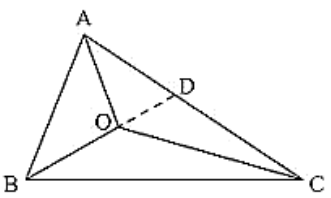We know that in a triangle the sum of any two sides is greater than the third side.

So, we have

In Δ ABC

AB + BC > AC

BC + AC > AB

AC + AB > BC

In ΔOBC

OB + OC > BC ... (i)

In ΔOAC

OA + OC > AC ... (ii)

In ΔOAB

OA + OB > AB ... (iii)

Now, extend (or) produce BO to meet AC in D.

Now, in ΔABD, we have

AB + AD > BO + OD ... (iv)  [BD = BO + OD]

Similarly in ΔODC, we have

OD + DC > OC ... (v)

(i) Adding (iv) and (v), we get

AB + AD + OD + DC > BO + OD + OC

AB + (AD + DC) > OB + OC

AB + AC > OB + OC ... (vi)

Similarly, we have

BC + BA > OA + OC ... (vii)

and CA+ CB > OA + OB ... (viii)

(ii) Adding equation (vi), (vii) and (viii), we get

AB + AC + BC + BA + CA + CB > OB + OC + OA + OC + OA + OB

⟹ 2AB + 2BC + 2CA > 2OA + 2OB + 2OC

⟹ 2(AB + BC + CA) > 2(OA + OB + OC)

⟹ AB + BC + CA > OA + OB + OC

(iii) Adding equations (i), (ii) and (iii)

OB + OC + OA + OC + OA + OB > BC + AC + AB

2OA + 2OB + 2OC > AB + BC + CA

We get = 2(OA + OB + OC) > AB + BC +CA

(OA + OB + OC) > (1/2)(AB + BC +CA)

Question: 6

Prove that the perimeter of a triangle is greater than the sum of its altitudes.

Solution:

Given, ΔABC in which AD ⊥ BC, BE ⊥ AC and CF ⊥ AB.

To prove,

AD + BE + CF < AB + BC + AC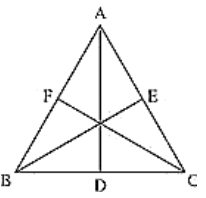Figure:

Proof:

We know that of all the segments that can be drawn to a given line, from a point not lying on it, the perpendicular distance i.e, the perpendicular line segment is the shortest.

Therefore

AB + AC > 2AD .... (i)

BE ⊥ AC

BA > BE and BC > BE

BA + BC > 2BE ... (ii)

CF ⊥ AB

CA > CF and CB > CF

CA + CB > 2CF ... (iii)

Adding (i), (ii) and (iii), we get

AB + AC + BA + BC + CA + CB > 2AD + 2BE + 2CF

2AB + 2BC + 2CA > 2(AD + BE + CF)

AB + BC + CA > AD + BE + CF

The perimeter of the triangle is greater than that the sum of its altitudes

Hence proved

Question: 7

In Fig., prove that: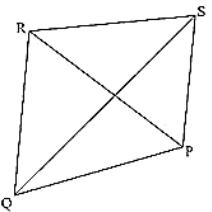(i) CD + DA + AB + BC > 2AC

(ii) CD + DA + AB > BC

Solution:

To prove

(i) CD + DA + AB + BC > 2AC

(ii) CD + DA+ AB > BC

From the given figure,

We know that, in a triangle sum of any two sides is greater than the third side

(i) So,

In ΔABC, we have

AB + BC > AC  .... (i)

CD + DA > AC .... (ii)

Adding (i) and (ii), we get

AB + BC + CD + DA > AC + AC

AB + BC + CD + DA > 2AC

(ii) Now, In Δ ABC, we have,

AB + AC > BC ... (iii)

CD + DA > AC

CD + DA + AB > AC + AB

From equation (iii) and (iv), we get,

CD + DA + AB > AC + AB > BC

CD + DA + AB > BC

Hence proved

Question: 8

Which of the following statements are true (T) and which are false (F)?

(i) Sum of the three sides of a triangle is less than the sum of its three altitudes.

(ii) Sum of any two sides of a triangle is greater than twice the median drown to the third side

(iii) Sum of any two sides of a triangle is greater than the third side.

(iv) Difference of any two sides of a triangle is equal to the third side.

(v) If two angles of a triangle are unequal, then the greater angle has the larger side opposite to it

(vi) Of all the line segments that can be drawn from a point to a line not containing it, the perpendicular line segment is the shortest one.

Solution:

(i) False (F)

Reason: Sum of these sides of a triangle is greater than sum of its three altitudes

(ii) True (T)

(iii) True (T)

(iv) False (F)

Reason: The difference of any two sides of a triangle is less than third side

(v) True (T)

Reason: The side opposite to greater angle is longer and smaller angle is shorter in a triangle

(vi) True (T).

Reason: The perpendicular distance is the shortest distance from a point to a line not containing it.

Question: 9

Fill in the blanks to make the following statements true.

(i) In a right triangle the hypotenuse is the ___ side.

(ii) The sum of three altitudes of a triangle is ___ than its perimeter.

(iii) The sum of any two sides of a triangle is ___ than the third side.

(iv) If two angles of a triangle are unequal, then the smaller angle has the ___ side opposite to it.

(v) Difference of any two sides of a triangle is ___ than the third side.

(vi) If two sides of a triangle are unequal, then the larger side has ___ angle opposite to it.

Solution:

(i) In a right triangle the hypotenuse is the largest side

Reason: Since a triangle can have only one right angle, other two angles must be less than 90

The right angle is the largest angle.

Hypotenuse is the largest side.

(ii) The sum of three altitudes of a triangle is less than its perimeter.

(iii) The sum of any two sides of a triangle is greater than the third side.

(iv) If two angles of a triangle are unequal, then the smaller angle has the smaller side opposite to it.

(v) Difference of any two sides of a triangle is less than the third side.

(vi) If two sides of a triangle are unequal, then the larger side has greater angle opposite to it.
```### Course Features

• 728 Video Lectures
• Revision Notes
• Previous Year Papers
• Mind Map
• Study Planner
• NCERT Solutions
• Discussion Forum
• Test paper with Video Solution